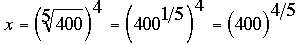SEARCH HOMEMath Central Quandaries & QueriesQuestion from Charles: I seem to remember you could solve exponential problems using natural logs quite simply without calculators, such as the fifth root of 400 or 33 to the 4th power. All you did was convert the number to its natural log and then just divide by 5 or multiply by 4 and then convert it back. I just remember how to do this. Thanks.Charles,

What you are remembering is that for any logarithm, log(ab) = b log(a). Thus for example ifthen log(4004/5) = 4/5 log(400). If you are using the natural log then

ln(x) = ln(4004/5) = 4/5 ln(400) = 4/5 × 5.99146 = 4.79317

and thus

x = eln(x) = e4.79317 = 120.6835

HarleyMath Central is supported by the University of Regina and The Pacific Institute for the Mathematical Sciences.